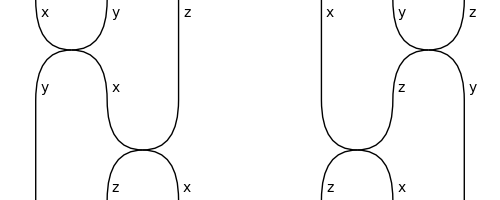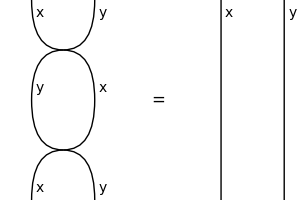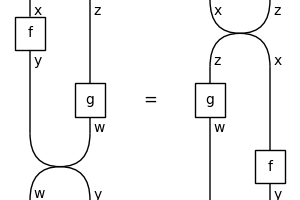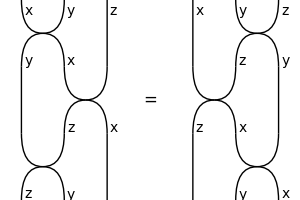# symmetric#

The free symmetric category, i.e. diagrams with swaps.

## Summary#

 `Diagram` A symmetric diagram is a balanced diagram with `Swap` boxes. `Box` A symmetric box is a balanced box in a symmetric diagram. `Swap` The swap of atomic types `left` and `right`. `Sum` A symmetric sum is a balanced sum and a symmetric box. `Category` A symmetric category is a balanced category with a method `swap`. `Functor` A symmetric functor is a monoidal functor that preserves swaps.

## Axioms#

```>>> from discopy.drawing import Equation
>>> Diagram.structure_preserving = True
>>> x, y, z, w = map(Ty, "xyzw")
>>> f, g = Box("f", x, y), Box("g", z, w)
```
• Triangle:

```>>> assert Diagram.swap(Ty(), x) == Id(x) == Diagram.swap(x, Ty())
```
• Hexagon:

```>>> assert Diagram.swap(x, y @ z) == Swap(x, y) @ z >> y @ Swap(x, z)
>>> assert Diagram.swap(x @ y, z) == x @ Swap(y, z) >> Swap(x, z) @ y
>>> Equation(Diagram.swap(x, y @ z), Diagram.swap(x @ y, z), symbol='').draw(
...     space=2, path='docs/_static/symmetric/hexagons.png', figsize=(5, 2))
```• Involution (a.k.a. Reidemeister move 2):

```>>> assert Swap(x, y)[::-1] == Swap(y, x)
>>> assert Swap(x, y) >> Swap(y, x) == Id(x @ y)
>>> Equation(Swap(x, y) >> Swap(y, x), Id(x @ y)).draw(
...     path='docs/_static/symmetric/inverse.png', figsize=(3, 2))
```• Naturality:

```>>> assert f @ g >> Swap(f.cod, g.cod) == Swap(f.dom, g.dom) >> g @ f
>>> Equation(f @ g >> Swap(f.cod, g.cod), Swap(f.dom, g.dom) >> g @ f).draw(
...     path='docs/_static/symmetric/naturality.png', figsize=(3, 2))
```• Yang-Baxter (a.k.a. Reidemeister move 3):

```>>> yang_baxter_left = Swap(x, y) @ z >> y @ Swap(x, z) >> Swap(y, z) @ x
>>> yang_baxter_right = x @ Swap(y, z) >> Swap(x, z) @ y >> z @ Swap(x, y)
>>> assert yang_baxter_left == yang_baxter_right
>>> Equation(yang_baxter_left, yang_baxter_right).draw(
...     path='docs/_static/symmetric/yang-baxter.png', figsize=(3, 2))
```
```>>> Diagram.structure_preserving = False
```